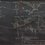# Polylogarithm recursion identity

I recently discovered a recursive identity for $\mathrm{Li}_{-m}(z)$ for $m$ a positive integer. Hopefully this will be of some use when tackling problems seeking for the value of some polylogarithmic sum.

$\mathrm{Li}_{-m}(z) = \frac{z}{1-z} \left[ 1+\sum_{k=0}^{m-1} \binom{m}{k} \mathrm{Li}_{-k}(z) \right]$

Proof:

Consider $\displaystyle \sum_{n=1}^{\infty} z^n(n+1)^m$. We have

\begin{aligned} \sum_{n=1}^{\infty} z^n(n+1)^m &= \sum_{n=1}^{\infty} z^n \sum_{k=0}^m \binom{m}{k} n^k \\ &= \sum_{n=1}^{\infty} z^nn^m + \sum_{k=0}^{m-1} \binom{m}{k} \sum_{n=1}^{\infty} z^nn^k \\ &= \mathrm{Li}_{-m}(z) + \sum_{k=0}^{m-1} \binom{m}{k} \mathrm{Li}_{-k}(z) \end{aligned}

We also have

\begin{aligned} \sum_{n=1}^{\infty} z^n(n+1)^m &= \frac{1}{z} \sum_{n=2}^{\infty} z^nn^m \\ &= \frac{1}{z} \mathrm{Li}_{-m}(z)-1 \end{aligned}

Equating the two gives

$\mathrm{Li}_{-m}(z) + \sum_{k=0}^{m-1} \binom{m}{k} \mathrm{Li}_{-k}(z) = \frac{1}{z} \mathrm{Li}_{-m}(z)-1$

When rearranged, we obtain

$\mathrm{Li}_{-m}(z) = \frac{z}{1-z} \left[ 1+\sum_{k=0}^{m-1} \binom{m}{k} \mathrm{Li}_{-k}(z) \right]$

as required.Note by Jake Lai
5 years, 5 months ago

This discussion board is a place to discuss our Daily Challenges and the math and science related to those challenges. Explanations are more than just a solution — they should explain the steps and thinking strategies that you used to obtain the solution. Comments should further the discussion of math and science.

When posting on Brilliant:

• Use the emojis to react to an explanation, whether you're congratulating a job well done , or just really confused .
• Ask specific questions about the challenge or the steps in somebody's explanation. Well-posed questions can add a lot to the discussion, but posting "I don't understand!" doesn't help anyone.
• Try to contribute something new to the discussion, whether it is an extension, generalization or other idea related to the challenge.
• Stay on topic — we're all here to learn more about math and science, not to hear about your favorite get-rich-quick scheme or current world events.

MarkdownAppears as
*italics* or _italics_ italics
**bold** or __bold__ bold
- bulleted- list
• bulleted
• list
1. numbered2. list
1. numbered
2. list
Note: you must add a full line of space before and after lists for them to show up correctly
paragraph 1paragraph 2

paragraph 1

paragraph 2

[example link](https://brilliant.org)example link
> This is a quote
This is a quote
    # I indented these lines
# 4 spaces, and now they show
# up as a code block.

print "hello world"
# I indented these lines
# 4 spaces, and now they show
# up as a code block.

print "hello world"
MathAppears as
Remember to wrap math in $$ ... $$ or $ ... $ to ensure proper formatting.
2 \times 3 $2 \times 3$
2^{34} $2^{34}$
a_{i-1} $a_{i-1}$
\frac{2}{3} $\frac{2}{3}$
\sqrt{2} $\sqrt{2}$
\sum_{i=1}^3 $\sum_{i=1}^3$
\sin \theta $\sin \theta$
\boxed{123} $\boxed{123}$

## Comments

Sort by:

Top Newest

A point to note is that $\operatorname{Li}_{-m} (z)$ , when $m$ is a positive integer, already has a closed form in terms of rational polynomial functions.

- 4 years, 11 months ago

Log in to reply

Similarly $\mathrm{Li}_{-m}(z)=\frac{\left(-1\right)^m}{1-z}\left[\sum _{k=0}^{m-1}\left(-1\right)^k\binom{m}{k}\mathrm{Li}_{-k}(z)\right]$

- 5 years, 5 months ago

Log in to reply

Nice!

- 5 years, 5 months ago

Log in to reply

×

Problem Loading...

Note Loading...

Set Loading...